# Sharing In A Ratio Worksheet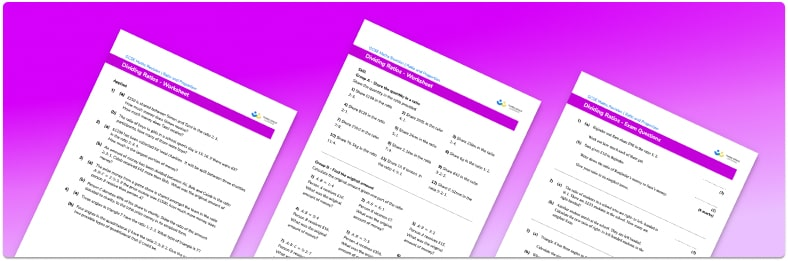• Section 1 of the sharing in a ratio worksheet contains 36 skills-based sharing in a ratio questions, in 3 groups to support differentiation
• Section 2 contains 4 applied sharing in a ratio questions with a mix of worded problems and deeper problem solving questions
• Section 3 contains 4 foundation and higher level GCSE exam style sharing in a ratio questions
• Answers and a mark scheme for all sharing in a ratio questions are provided
• Questions follow variation theory with plenty of opportunities for students to work independently at their own level
• All questions created by fully qualified expert secondary maths teachers
• Suitable for GCSE maths revision for AQA, OCR and Edexcel exam boards

• This field is for validation purposes and should be left unchanged.

You can unsubscribe at any time (each email we send will contain an easy way to unsubscribe). To find out more about how we use your data, see our privacy policy.

### Sharing in a ratio at a glance

A part to part ratio is used to share a a quantity into a given ratio. The quantity is divided by the sum of the parts of the ratio and then multiplied by each part of the ratio to give the solution. Each part is therefore a proportion of the total amount.

Sometimes writing a ratio in its simplest form can make it easier to divide a quantity into a ratio as we are using an equivalent ratio but the integers are more manageable to work with.

Looking forward, students can then progress to additional ratio worksheets and other ratio and proportion worksheets, for example a speed distance time worksheet or a direct proportion worksheet.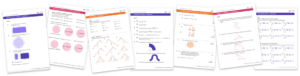For more teaching and learning support on Ratio and Proportion our GCSE maths lessons provide step by step support for all GCSE maths concepts.

## Related worksheets

Ratio To Fraction Worksheet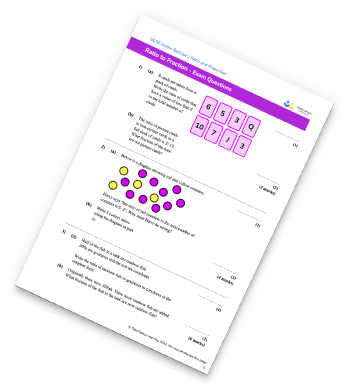Simplifying Ratios Worksheet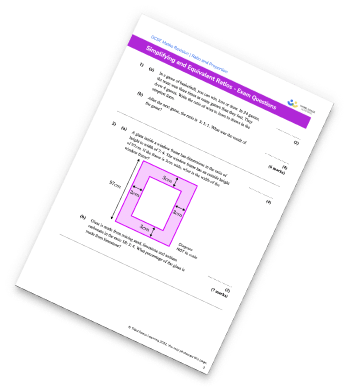Ratio Worksheet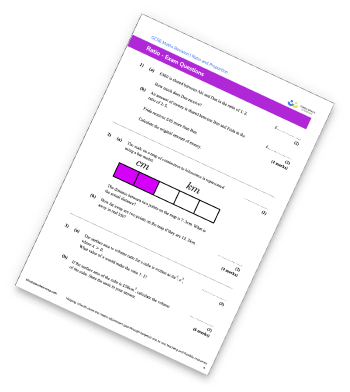Density Worksheet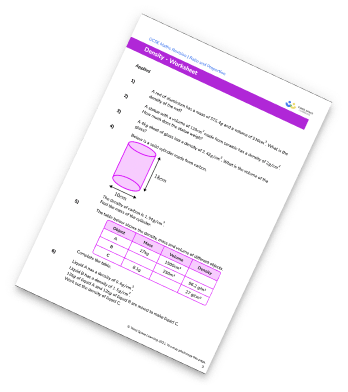## Do you have KS4 students who need more focused attention to succeed at GCSE?There will be students in your class who require individual attention to help them succeed in their maths GCSEs. In a class of 30, it’s not always easy to provide.

Help your students feel confident with exam-style questions and the strategies they’ll need to answer them correctly with our dedicated GCSE maths revision programme.

Lessons are selected to provide support where each student needs it most, and specially-trained GCSE maths tutors adapt the pitch and pace of each lesson. This ensures a personalised revision programme that raises grades and boosts confidence.

Find out more